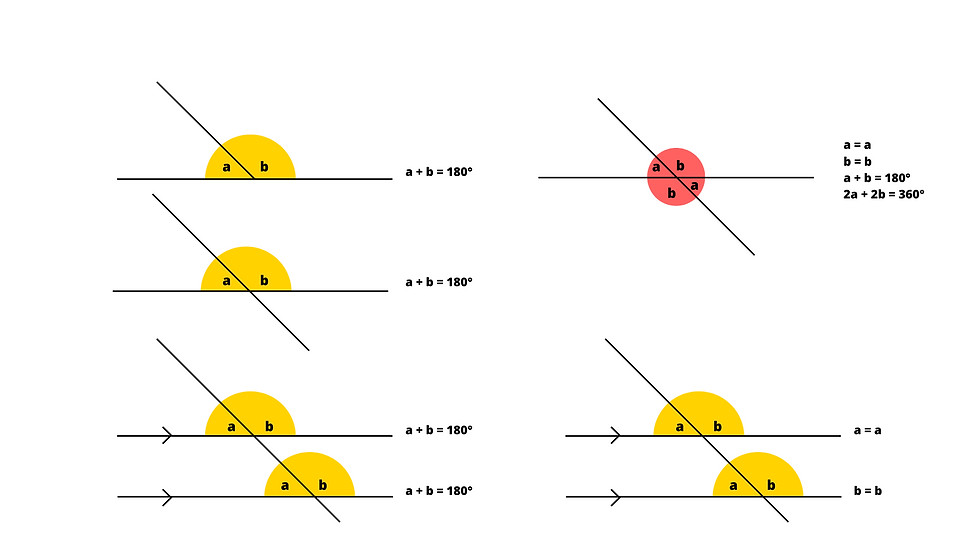top of page
Search

# How I wish I'd taught... angles.

Updated: Feb 24

What is an angle?

An angle is defined as the measure of rotation of one line or plane relative to another, usually measured in degrees. It is formed by two rays with a common endpoint, called the vertex of the angle. An angle can also be described as the amount of turn between two lines or planes.Types of angles

Acute -

An acute angle is an angle that measures less than 90 degrees. It is an angle that is smaller than a right angle. The name "acute" comes from the Latin word "acutus", which means "sharp" or "pointed", which describes the shape of an acute angle. An acute angle is often represented by the symbol ∠ and is measured in degrees (°).

Right angle -

A right angle is an angle that measures exactly 90 degrees. It is formed when two lines or segments meet at a 90-degree angle, or when a line or segment is perpendicular to another. A right angle can also be described as a quarter-turn or a 90-degree rotation. The symbol for a right angle is a small square (▭) at the vertex of the angle and it is often used in geometry.

Obtuse angle -

An obtuse angle is an angle that measures greater than 90 degrees but less than 180 degrees. It is an angle that is larger than a right angle but smaller than a straight angle. The name "obtuse" comes from the Latin word "obtusus", which means "blunt" or "dull", describing the shape of an obtuse angle.

Straight angle -

A straight angle is an angle that measures exactly 180 degrees. It is formed when two rays point in exactly opposite direction . It is also referred as a "flattened" angle or "half-rotation" angle. It can be considered as the sum of two angles that are opposite rays.

Reflex angle -

A reflex angle is an angle that measures greater than 180 degrees but less than 360 degrees. It is formed when a line or segment rotates more than a half-turn but less than a full turn. The name "reflex" comes from the Latin word "reflectere" which means "to bend back" or "to turn back", describing the shape of a reflex angle. It is also known as a "concave angle"

Full angle -

A full angle is an angle that measures exactly 360 degrees. It is formed when a line or segment completes a full rotation or one full turn around a point. It can also be thought of as the sum of all four right angles. A full angle is also called a "Perigon" or "Revolution" angle. In geometry, a full angle is a way of measuring the size of an angle that goes around the whole circle.

Common symbols.

Lines of equal length are represented using a single dash. If there are two equal sized lines, but the set is different, then double dashes are used.

Lines that are parallel are shown using a single arrow. Double arrows are used to differentiate between two different pairs of parallel lines.

Angles that are identification are shown using a single dash through the angle or by using a pair of curved lines.Triangles.

As the name suggests, triangles have 3 angles. Tri meaning 3 and angles meaning the number of verticies within the shape. An important fact to retrieve is that of the interior angles of a triangle add to the sum of 180°. You could model this in class using the following:

• Draw a triangle using exact measurements stated by the teacher.

• Mark the angles in a triangle.

• Tear the angles and rearrange them to show them on a straight line, asking what the children notice.Angles on lines.

Now children have made connections between triangles and straight lines, we may use the fact to develop a depth of thinking. Teaching children to recognise equivalent angles on lines will allow them to reason deeply about their understanding.